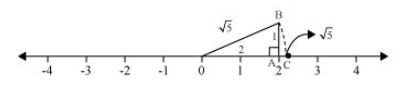# Show how √5 can be represented on the number line.

Solution:

We know that, $\sqrt{4}=2$

And, $\sqrt{5}=\sqrt{(2)^{2}+(1)^{2}}$Mark a point ‘A’ representing 2 on number line. Now, construct AB of unit length perpendicular to OA. Then, taking O as centre and OB as radius, draw an arc intersecting number line at C.

$C$ is representing $\sqrt{5}$.